/

### Function Description

Returns the de-smoothing parameter corresponding to the AR(1) weighted de-smoothed (or ‘de-correlated’) values of a series, as described in the book Extreme Events. This involves:

(a)    Postulating (for, say, a return series) that there is some underlying ‘true’ return series,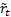, and that the observed series,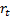, derives from it via a first order autoregressive model,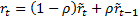; and

(b)   Identifying the value of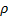(between 0 and 0.5) for whichhas zero weighted first order correlation (which implies that the covariance between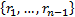and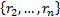is zero.

The de-smoothed series,, can then be derived using the following formula, see MnWeightedDesmooth_AR1: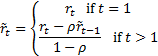In step (b) we weight the elements in the covariance computation using weights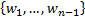(where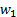corresponds to the weight given to the component arising from the term in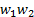). If the weights are equal then returns the same as MnDesmooth_AR1_rho.

Contents | Prev | Next